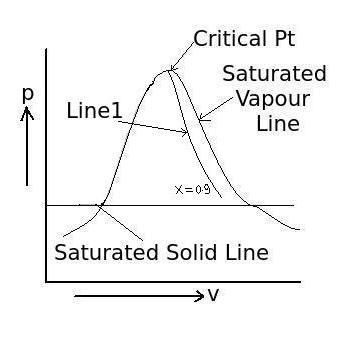# Quality and Dryness Fraction - MCQs with Answers

Q1. If m1 and m2 are the masses of liquid and vapour respectively in a liquid-vapour mixture, then what is the formula for dryness fraction x?

a. x = (m1 + m2) / m1
b. x = (m1 + m2) / m2
c. x = m1 / (m1 + m2)
d. x = m2 / (m1 + m2)

ANSWER: d. x = m2 / (m1 + m2)

Q2. What is the dryness fraction (x) for saturated water, when water just starts boiling?

a. x = 0
b. x = 1
c. x = 0.9
d. x = 0.5

Q3. What is the dryness fraction (x) on saturated vapour line?

a. x = 0
b. x = 1
c. x = 0.9
d. x = 0.5

Q4. What is the line which starts from critical point having constant dryness fraction throughout the line as shown in figure (Line1) called?a. constant vapour line
b. constant liquid line
c. constant quality line
d. none of the above

Q5. The dryness (x) fraction of superheated steam is taken as

a. x= 0
b. x= 0.9
c. x= 0.999
d. x= 1

Q6. What is a liquid, whose temperature is less than the saturation temperature at the given pressure called?

a. compressed liquid
b. subcooled liquid
c. both a. an b.
d. none of the above

ANSWER: c. both a. an b.

Q7. What is the degree of subcooling?

a. the difference between saturation temperature of liquid and actual temperature of liquid
b. the difference between saturation temperature of vapour and actual temperature of liquid
c. the difference between saturation temperature of liquid and actual temperature of vapour
d. the difference between saturation temperature of vapour and actual temperature of vapour